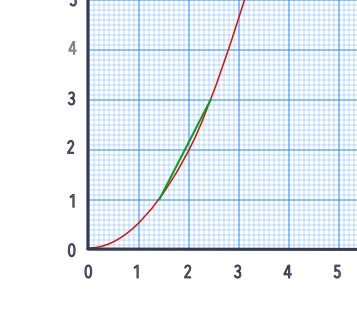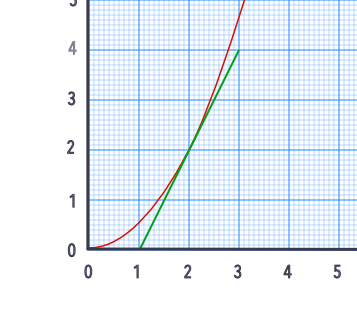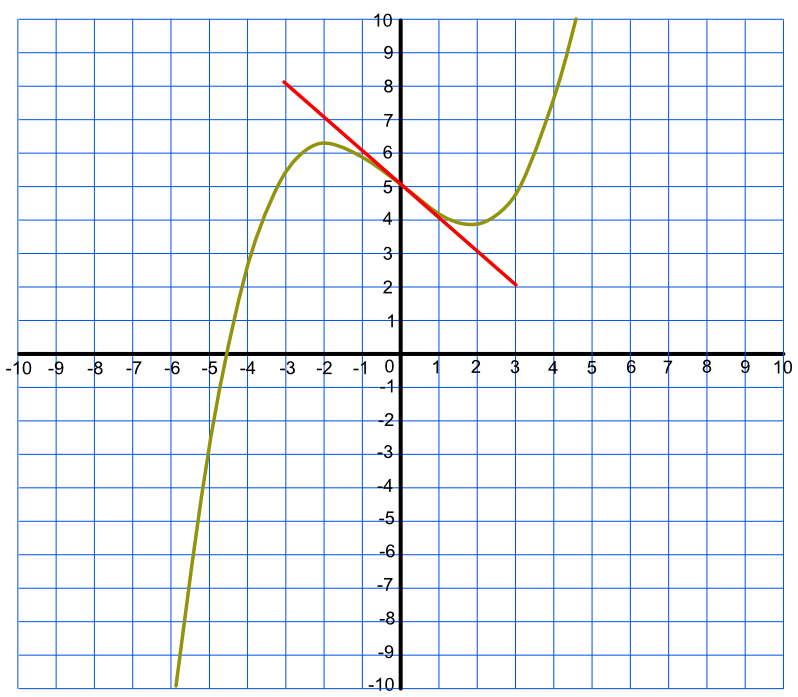Gradient at a Point

# Gradient at a Point

GCSE(H),

The gradient of a graph indicates the rate of change. In the example below, the rate of change of y with respect to x (in other words, how y is changing when x is changing) increases as the value of x increases.

The gradient of a graph can be obtained by two methods. An average rate of change can be obtained by drawing a chord over the point. The second method is to take a tangent at the point, and to determine the gradient of the tangent.In this instance, the average (chord) method gives the same answer as the instantaneous (tangent) method, but the average method will be unsuitable for graphs with turning points.

## Examples

1. What is the average gradient for the function y = 3x^3 -x + 5 at x = 0? Use the points between x=-3 and x=+3?

y coordinate for x=-3 is y = 5.3 and for x=+3 is y=4.7
The gradient = frac(4.7 - 5.3)(3 - -3) = frac(-0.6)(6) = -0.1
2.What is the instantaneous rate of change for the function y = 3x^3 -x + 5 when x=0? The tangent has been drawn for you, and the end points of the tangent are (-3, 8) and (3, 2).
The gradient is frac(text(change in )y)(text(change in ) x) = frac(2 - 8)(3 - -3) = frac(-6)(6) = -1